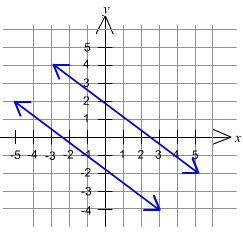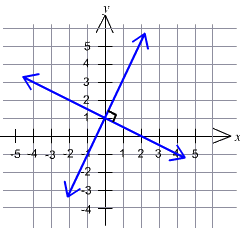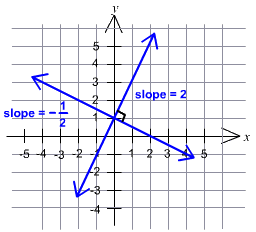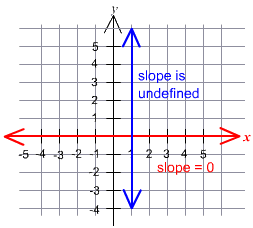## Describe about parallel and perpendicular lines, Mathematics

Assignment Help:

Describe about Parallel and Perpendicular Lines ?

Parallel Lines : Parallel lines are coplanar lines (lines that lie in the same plane) that never intersect.The blue lines are parallel. Notice that these lines have the same slope.
Two lines with the same slope are parallel.
If they had different slope then they would intersect and so they wouldn't be parallel.
If two no vertical lines are parallel, they have the same slope.
What if the lines are vertical?
If two vertical lines are parallel, they both have undefined slope.
(See example 2 for more on horizontal and vertical lines.)
Perpendicular Lines
Perpendicular lines intersect at an angle of 90 degrees, in other words they form a right angle.How do the slopes of these perpendicular lines compare to each other?The slopes of the two perpendicular lines are 2 and -1/2. How are these slopes related? -1/2 is the negative reciprocal of 2. (See the basics section for more about negative reciprocals.)
If two lines are perpendicular, their slopes are negative reciprocals.If the slopes of two lines are negative reciprocals, then the lines are perpendicular.

Example 1: What is the slope of a line that is
a. parallel to 12x - 3y = 10?
b. perpendicular to 12x - 3y = 10?
Solution: First let's find the slope of the line 12x - 3y = 10.
Put the equation in the form y = mx + b.
12x - 3y = 10
-3y = -12x +10
-3y/-3 = -12x/-3 +10/-3
Y= -4x -10/3
The slope of the line is -4.
a. All lines that are parallel to 12x - 3y = 10 have slope equal to this line. The slope of a line parallel to 12x - 3y = 10 is -4.
b. All lines that are perpendicular to 12x - 3y = 10 have slope equal to the negative reciprocal of -4. The negative reciprocal of -4 is 1/4, so the slope of a line perpendicular to 12x - 3y = 10 is 1/4.

Example 2: What is the slope of a line that is
a. parallel to the x'axis?
b. perpendicular to the x'axis?
Solution: The x'axis is a horizontal line. All horizontal lines have zero slope.
a. A line parallel to the x'axis has slope = 0.
b. A line perpendicular to the x'axis is vertical. All vertical lines have undefined slope.

#### Recursively, Let a 0 , a 1 ::: be the series recursively defined by a 0 =...

Let a 0 , a 1 ::: be the series recursively defined by a 0 = 1, and an = 3 + a n-1 for n ≥ 1. (a) Compute a 1 , a 2 , a 3 and a 4 . (b) Compute a formula for an, n ≥ 0.

-10b2*-5b2=

#### Can you explain slope, Can you explain slope and Slope is measured as rise/...

Can you explain slope and Slope is measured as rise/run?

#### Optimization, Optimization : In this section we will learn optimization p...

Optimization : In this section we will learn optimization problems.  In optimization problems we will see for the largest value or the smallest value which a function can take.

#### Topology, Is usual topology on R is comparable to lower limit topology on R...

Is usual topology on R is comparable to lower limit topology on R

#### Diffrence between integers and rational numbers, Q. Give basic Diffrence be...

Q. Give basic Diffrence between Integers and Rational Numbers? Ans. Integers The integers are positive and negative whole numbers. The integers are closed under ad

#### Differential calculus, lim n tends to infintiy ( {x} + {2x} + {3x}..... +{n...

lim n tends to infintiy ( {x} + {2x} + {3x}..... +{nx}/ n2(to the square) )where {X} denotes the fractional part of x? Ans) all no.s are positive or 0. so limit is either positive

#### first and third quartiles, From the data given below calculate the value o...

From the data given below calculate the value of first and third quartiles, second and ninth deciles and forty-fifth and fifty-seventh percentiles.

#### How much does every person required to pay rob, Rob purchased picnic food f...

Rob purchased picnic food for \$33.20 to share along with three of his friends. They plan to split the cost evenly among the four friends. How much does every person required to pay